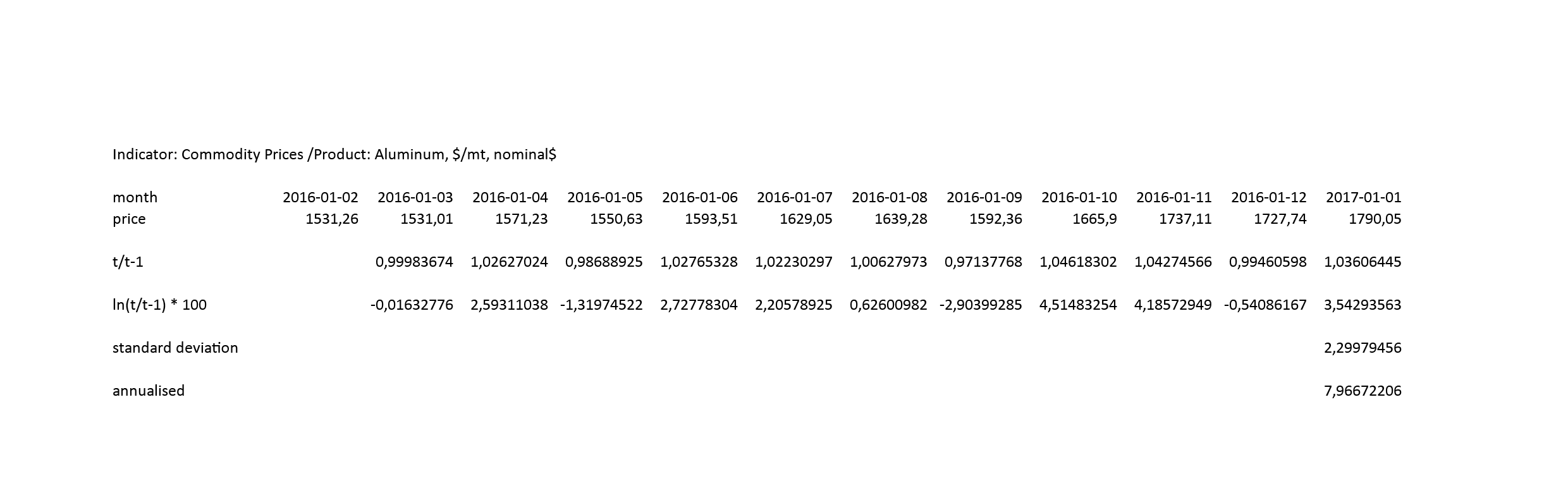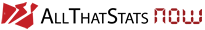# Definition

Volatility is a statistical value measuring the amount of uncertainty or risk faced by participants in financial markets: e.g. the size of changes in security values, commodity prices or corresponding indexes.

Volatility can be measured by calculating the standard deviation or variance from the past variations of the asset prices:

• The higher the volatility, the higher the risk that an asset price can change dramatically over a short time period in either direction.
• The lower the volatility, the asset price is not to be expected to fluctuate dramatically, but changes in value may occur at a steady pace over a period of time.

# Math Example

### Indicator: Commodity Prices /Product: Aluminum, \$/mt, nominal\$Here, we found a nice video tutorial at study.com referring to calculating price volatility.

All graphics and tables are created with